# The Geometric Viewpoint

geometric and topological excursions by and for undergraduates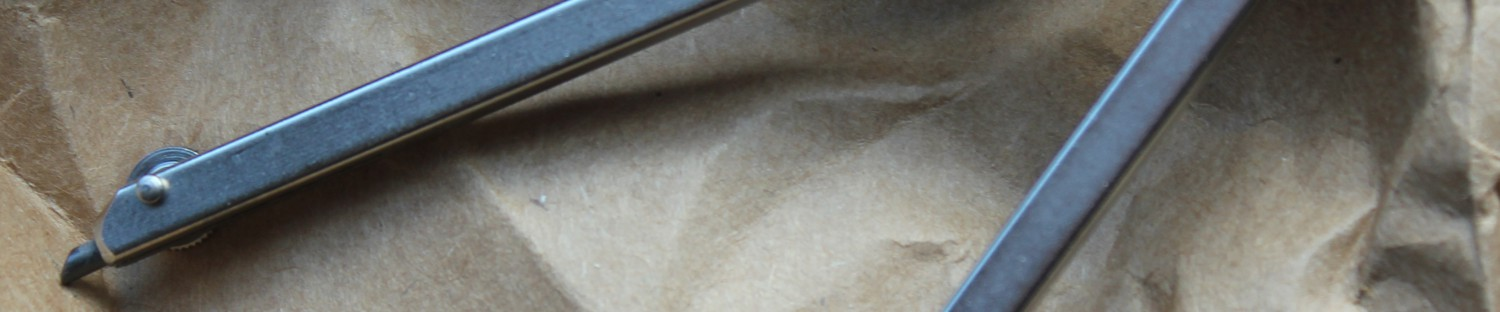## Algorithms for Working with Triangulated Surfaces

Let’s imagine that you and your friends just took out a steaming pepperoni pizza out of the oven. How would you go about triangulating (divide using triangles) the surface (the pizza) into equal pieces? This shouldn’t be to hard, should it? But what if someone took a knife and placed a couple of points on the pizza, and said the triangulation has to contain triangles that have those points as vertices. How would unite the dots, making sure that the triangles you get yield the fairest division? Not so easy is it?

This is a problem that most graphics software engineers encounter. But their conundrum is even more complicated as they deal with millions of points located in 3D space, and the process they’re using has to be automated and work for every possible case. Because we will use this problem to guide us through the concepts presented in this post, we should first offer a clear description of the problem and of the solution we are looking for. In Computer Graphics, models (let’s say a human face) are made of thousand of patches that consist of a fixed number of points (usually numbers that are perfect squares). These points keep track of their position in 3D space (these are called world coordinates, as they lie in the coordinate system of the model). Because the computer programmer wants to create a realistic human face while making his program fast, he or she devises an algorithm that subdivides the patches into subpatches that have less than a certain distance in screen coordinates between adjacent points (i.e. the distance between two points on the screen will be smaller than an certain limit). This way, instead of having nice patches made out of 16, 25 or 36 points, we end up with all sorts of random configuration of points. And we have lots of such configurations. We are interested in finding an algorithm that, after the aforementioned subdivision, is able to create a triangulation of all the points in the model, while rendering an image as realistic as possible. By realistic, we understand that we don’t want our triangles to be too skinny, too big or too small, because that would create a deformed face (not that every face isn’t beautiful in its own way). Also, we are not allowed to have edges crossing each other (because that wouldn’t be a triangulation anymore), and we are not allowed to add new points.  To keep the problem simple, we will ignore things like aliasing and hidden surface removal, and we will also reduce our example to two dimensions. The presented method works in all dimensions, and the problem can actually be reduced to two dimensions by creating a bounding cube around the object and reflecting the points onto the closest face).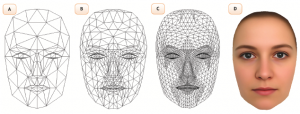This is the kind of result we are looking for. Note that in this case, a different type of subdividing from the one we presented is used. The points are more dense in regions were the protuberances of the faces are more pronounced.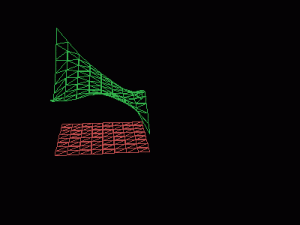This is how we can reduce the problem from three dimensions to only two dimensions.

A mathematical structure that encompasses all of the above requirements is the Delaunay Triangulation. Before jumping into any algorithms, we shall first try to gain a mathematical understanding of Delaunay Triangulations. We can define Delaunay Trinagulations in two ways:

• Geometrically,  a Delaunay triangulation for a set P of points in a plane is a triangulation DT(P) such that no point in P is inside the circum-circle (the unique circle that passes through each of the triangle’s three vertices) of any triangle in DT(P) .
• Topologically, a Delaunay triangulation of a ﬁnite set${{S}\subseteq \mathbb{R}^n}$ is isomorphic to the nerve of the Voronoi diagram of${{S}}$.

As with most things in life, let us first ignore the harder definition and focus on the geometric one. The following image should make the definition clearer: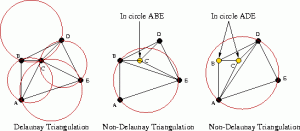As you can see, the first picture is a Delaunay Triangulation because there are no points within any of the four circumcircles.

Because for our set${{S} = \left\{ {A,B,C,D,E}\right\} }$ no points lie within the circumcirlces of triangles${\Delta ABC,\Delta ACE,\Delta CED,\Delta BCD}$, then${{DT(P)} = \left\{\Delta ABC,\Delta ACE,\Delta CED,\Delta BCD\right\}}$. Note that the triangulation of a set of points is a set of triangles. This definition is clear and easy, and it will be the one we will use when implementing our algorithm. However, why this specific triangulation works for our imposed conditions is not that apparent. If we take a look at the three triangulations above, we can observe that the Delaunay Triangulation is the most ‘aesthetically pleasing’ one, in the way that we don’t have long, slim triangles and that we only connect points that are close to each other. As it turns out, this ‘aesthetically pleasing’ property is given by the fact that Delaunay Triangulations maximize the minimal angle. In other words, the minimal angle of all the triangles in a Delaunay Triangulation of a set${{P}}$ , will be larger than the minimal angle of all the other triangulations of${{P}}$. Why does the property that no point of${{P}}$ lies within the circumcircle of any triangle maximize the minimal angle? Pick a triangle in our triangulation and look at its least angle. Observe that as we move the vertex at that angle away from the center of the circle, two things happen: the circle increases in area along with the odds of another point of the set entering the circle, and the angle further decreases. So, because we are trying to form triangles with circumcircles that are as small as possible (ATTENTION: this is only an implication that is generally true, but it isn’t by any means a requirement, as Delunay triangulations don’t concern minimizing the edge length),we are ensuring that our triangulation connects points that are close and that we are avoiding ‘ugly’ triangles. This makes the Delaunay triangulation a perfect solution to our problem. Other properties of Delaunay Triangulations that we will take for granted are: every set of points in the Euclidean Space has a Delaunay Triangulation that is unique if no two points lie on the same sphere, the union of all the triangles form the convex hull of the set of points (i.e. the minimum convex set containing all the points in the set or a rubber band stretched using the points of the set so that it contains all of the points in the set in its interior).

Now let’s turn our attention towards the topological definition of a Delaunay Triangulation. At a first glance, the definition seems discouraging, but as we will set out to explain the terms, we will see that the sky isn’t that gray. The first word of the day is ‘isomorphic’, which for all intents and purposes means ‘the same’. The second math term is ‘nerve’. Lets look at the picture below and try to make sense of it:

Given a union of triangles${{T_i}}$, we can form its nerve${{N}}$ by following these steps. Start with${{N}}$ empty, and for each triangle in the union associate one point${{t_i}}$ and add it to${{N}}$. Then, look at non-empty intersections of the triangles(non-empty means that they share at least a point), and for every point shared by at least two triangles add a new set formed with the${{t_i}}$‘s corresponding to the triangles that share that point. In this process you cannot repeat sets. If you correctly cover all the possible intersections,${{N}}$ will be the nerve of the union of triangles. Observe that${{N}}$ is a set containing sets of one or more points, sets which we can graphically represent through points, edges and polygons as seen in the picture above. The final unknown term is “Voronoi diagram”. Again, pictures comes in handy: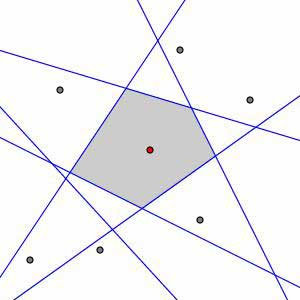We form the Vornoi cell of a point P by taking the intersection of all the half-spaces that contain P.

The Voronoi diagram(the whole first picture) of a set of points(the black points)${{S}\subseteq \mathbb{R}^n}$ is the collection of all the Voronoi cells(the colored polygons), denoted by${{V_u}}$, where${{u}}$ is a point of${{S}}$ and${{V_u} = \left\{x\in\mathbb{R}^n\mid \|x-u\| \le \|x-v\|,v\in\mathbb{R}^n\right\} }$. This definition tells us that every point in the cell${{V_u}}$ is closer to${{u}}$ than it is to any other point in${{S}}$ and that the points on the edges of the diagram are equally distanced from the points that generate incident Voronoi cells.  So, using this definition, we can form the Voronoi cell of a point${u\in{S}}$ by dividing the plane, for each other point${v\in{S}}$, into two pieces(a.k.a. ‘half-spaces’) using lines that are equally far away from${{u}}$ and${{v}}$. Then, if we take the intersection of all the half-spaces that contain${{u}}$, we will end up with${{V_u}}$. Although the definition allows for building Voronoi diagrams for spaces with dimension greater than 2, it turns out that for computational purposes this is not feasible process, as it a huge amount of time and storage space.

To plunge even deeper into topology, we present two more constructions for the Voronoi cells. To do this, we will go down one more dimension to create some visual intuition of the constructions, and then let the reader work his newly gained knowledge by extending the example to two dimensions. Use the picture bellow for reference. The Voronoi Diagram of a set${{S}\subseteq \mathbb{R}^1}$ is nothing more than a segmented line, where the segments are the Voronoi cells(in our drawing, the Voronoi diagram of the set${{S} = \left\{ {a,b,c,d}\right\} }$ is the segmented equator). We will lift our Vornoi cells to two dimensions by using stereographic projection. Intuitively, the stereographic projection of circle(sphere for two dimensions) is the shadow of its points cast by a single light on the surface of the circle onto a line(plane in two dimensions). By casting shadows, we mean picking a point${{N}}$ and drawing lines from it that intersect both a point on the circle and the line . This creates a mapping( an isomorphism, if you will), between the circle minus the point${{N}}$ and the line. So, we draw a circle that has our space as its equator, and take the north pole as our point${{N}}$. Using the inverse of the stereographic projection of the circle(i.e. we start from points on our space and draw lines that go through${{N}}$ and intersect the circle(the brown lines in our drawing)), we map our set${{S} = \left\{ {a,b,c,d}\right\} }$ onto the circle. For the first construction, we will draw tangent lines to the circle at the newly obtained points. These are the Pa,Pb,Pc,Pd in our drawing. Look carefully at their intersections. It seems that if we were to draw a line connecting the intersecting points and${{N}}$, we would intersect the line at the boundary of the Voronoi cells of the points that yielded the tangent lines. This turns out to be true. It is also true that if we draw lines from${{N}}$ to the stereographic projection of the points in our space, the line which we will first intersect, corresponds to the Voronoi cell to which the point belongs. For our second construction, we can build circles(Ca,Cb,Cc,Cd) that contain both${{N}}$ and the points in${{S}}$ and that are tangent to the equator. Take a moment to convince yourself that these circles are unique. Again look at the points of the intersections of the circles and remark that a line containing${{N}}$ and any of these points will also contain the boundary of two Voronoi cells. Thus, the first intersection of the segment connecting a point in our space and${{N}}$ is with the circle corresponding to the Voronoi cell to which the point belongs.

Having our terms all sorted out, it becomes apparent what the second definition means. Broadly, it tells us that by drawing edges between the points that generate two neighboring Voronoi cells, we will get a Delaunay Triangulation of the set of points. Observe that the edges we are drawing to geometrically represent the nerve of the Voronoi diagram are always perpendicular to the boundaries of the cells. Just try for a moment to draw a Voronoi diagram that yields triangles with small angles, and you will begin to see why this property maximizes the minimal angle. The only problem would be having more than 4 cells meeting at a point. As it turns out, this is equivalent to four points lying on the same circle, which, as we’ve stated in the first part, makes for having more than one Delaunay Triangulation.

These constructions are less intuitive and you might ask why do we need them when we already have something as simple as the first definition of a Delaunay Triangulation. The reason is that there exist algorithms such as Fortune’s Algorithm or Bowyer-Watson Algorithm that generate Delaunay Triangulations using Voronoi cells. These algorithms prove to be more useful as they can create Vornoi diagrams which have multiple applications across a vast array of domains.

Now that we have a strong theoretical basis, let us focus on one algorithm for creating a Delaunay Triangulation. Note that there are a lot of algorithms for doing this, but the one that we will be presenting is one of the simplest and most time efficient. The algorithm that we choose to implement employs the “divide and conquer” strategy.

Let us start with a set of random points ( we will work with an example that has 12 points to gain a better intuition). We begin by ordering them with respect to their x-coordinate. Also, if two points have the same x-coordinate, their ordering is determined by their y-coordinate in increasing order.

We continue by subdividing the points into halves using their labeling, until we are left with sets of two or three points. If we can’t split a set into two equal sets, we let the first subset to have one more element then the second. We then triangulate the newly formed sets by creating triangles in the case where we are left with three vertices, and edges in the case where we are left with two vertices.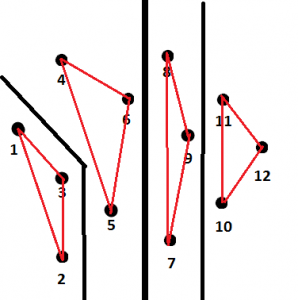The way we divide the set of points and the triangulations we can form. The thickness of the black lines indicates the order in which we divide starting from thickest

By taking a look at the picture, we can observe that we obtain a bunch of triangles with sharp angles which should give us an idea that most of the triangles formed in these first steps are not part of the Delaunay triangulation. However, as we have discussed earlier, the triangles in a Delaunay triangulation tend to be small, so it is very likely that some of the edges coincide. For a large number of vertices, this a huge time saver.

The next step is to merge neighboring sets of points by adding edges in the opposite order in which we have divided them. We will use the following notation to distinguish between edges: LL are edges that were formed using vertices from the left division, RR are edges that were formed using vertices from the right division, and LR are edges that were formed using vertices from both. We will try to now merge the first two divisions. For now we only have LL and RR edges. Throughout the process we will delete LL and RR edges but never add more, and we will try to add LR edges that are as good as possible. Remember that union of the triangles in a Delaunay triangulation of a set of points forms the convex hull of that set of points. Thus, we can safely assume that our first LR edge, which will certainly be in the final triangulation, is the edge between the two points with the smallest y-coordinate of the two divisions. Observe that this is also true for the upper part of the set of points.

Now it’s time to look for the next LR edge that can only be added above the previously created LR. To get a triangulation, we need this edge to start at one of the vertices of the previously added edge. Thus, we are looking for an vertex that forms with the previous LR edge a triangle whose circumcircle does not contain any other points. By the existence of a Delaunay triangulation and by its uniqueness(if we don’t have four co-circular points) we are guaranteed that such a point exists and that it is unique. We will look at the possible candidates and pick the best one from each side and then the best one from the two. Let us start with the right side. We will look at the vertices that share an edge with point 5 and pick the ones that form the two smallest angles with the previously added LR edge. The vertex associated with the smallest angle will be the Current Potential Candidate(CPC) and the other vertex will be the Next Potential Candidate(NPC). If our CPC fullfils the two following criteria we found our candidate for the right side :

• The clockwise angle from the base LR-edge to the potential candidate must be less than 180 degrees. (This ensures that we have traingles and that we don’t go outside the convex hull)
• The circumcircle defined by the two endpoints of the base LR-edge and the potential candidate must not contain the next potential candidate in its interior. (This is the Dealunay criterion that no circumcircle contains a point in its interior)

If our CPC doesn’t fullfill the criteria, we set CPC to NPC and then get the next NPC by looking at the next vertex in order of the angle mentioned above. We repeat this process until we either find a good candidate or we run out of candidates. If we do run out of candidates, the best candidate from the other side will win. If neither side has a candidate, then our merge is complete.

The process for the right side is similar, but instead of point 5 we use point 2 and we measure the angle at point 2 counter-clockwise. If we candidates from both sides, we repeat the circle test and pick the point for which the other point is not in the circumcircle. Be the existence of the Delaunay triangulation, we are guaranteed that such a point exists.

Once we get a “winner” vertex, we delete any vertex that intersects the newly formed LR edge.

We repeat this process until we have completed the merge, then move on through all the merges until our triangulation is complete. Note that any step of the way, we can delete previously added edges, so don’t assume that any of the created LR edges will be part of the final triangulation.

I will display the process for the first merges and then just display the result of the second and third merges: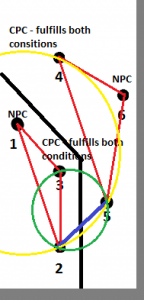Vertex 3 and vertex 4 are the candidates from each side, but vertex three obviously wins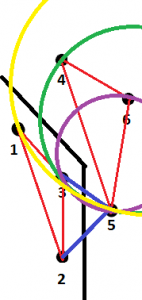Right side:
We start with 4 but fail the test so 6 is selected.
Left side: we can’t pick 2 because the angle is more than 180, but 1 works.
Of the two candidates 6 wins.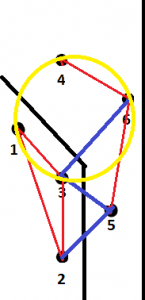Observe that we have 4 co-circular points, so we can pick either 34 or 16 as our edge

Triangulations seem like an easy mathematical concept, but things get a lot more complicated when you only have simple instructions at your disposal. Next time you want a brain teaser, try to forget everything you have just read, and attempt to create an algorithm that triangulates ( not even a Delaunay Triangulation) the convex hull of a set of points. You will see how things that you can easily do with a pen and a piece of paper become a lot harder to translate into instructions that work for all cases and that a machine can understand. That’s why computer science requires a vast knowldge  of mathematical concepts that are able to describe the same object or process into different modes, allowing the programmer to pick a method that can be transformed efficiently into code.

This entry was posted in Uncategorized. Bookmark the permalink.